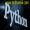# Series下标索引、标签索引、切片索引、布尔索引老董2020-11-17161围观,100赞

Series的values属性可以批量获取值，而利用Series的索引获取值更加常用且灵活。

Series是dict-like类型，也是list-like类型，可以模仿字典(比如可以用get方法获取数据)和列表(比如切片)获取数据。Series索引可以分为位置下标、标签索引、切片索引、布尔型索引。，。

下标索引

1、列表可以用负值如list[-1]，Series下标索引不能用负数。

2、多个标签需要传入列表，且结果是新的Series。

```# -*- coding: utf-8 -*-

import pandas as pd
import numpy as np

# 下标索引
arr = np.random.rand(5)
s = pd.Series(arr)
print(s)
print('------------')
print(s)
print('=====')
print(s[[0,3]])

```
```0    0.335132
1    0.037658
2    0.008121
3    0.576139
4    0.669062
dtype: float64
------------
0.33513248567621046
=====
0    0.335132
3    0.576139
dtype: float64
[Finished in 1.8s]

```

标签索引

1、Series的index参数就代表标签索引，标签索引和下标索引同时存在。

2、index最好用字符串否则视觉上不易分辨是下标索引还是标签

3、选取多个标签需要传入列表，且结果是新的Series

```# -*- coding: utf-8 -*-

import pandas as pd
import numpy as np

dic = {'语文':100,'数学':90,'英语':95}
s = pd.Series(dic,name='成绩')
print(s)
print('------------')
print(s['语文']) # 语文成绩
print('---------------')
print(s[['语文','数学']]) # 传入列表,多个标签
```
```语文    100

Name: 成绩, dtype: int64
------------
100
---------------

Name: 成绩, dtype: int64

```

切片索引

1、用下标切片就是左闭右开(末端不包含)。

2、用标签切片就是末端包含。

```# -*- coding: utf-8 -*-

import pandas as pd
import numpy as np

# 切片索引
s = pd.Series([1,2,3,4,5], index=['a', 'b', 'c', 'd', 'e'])
print(s)
print('-------')
print(s[0:2])
print('--------')
print(s['a':'c'])

```
```a    1
b    2
c    3
d    4
e    5
dtype: int64
-------
a    1
b    2
dtype: int64
--------
a    1
b    2
c    3
dtype: int64

```

布尔型索引(用来筛选数据)

布尔索引就是可以直接传入True、False这种值来选择数据，传入的类型可以是列表(元素是Ture和False)、Series、np数组，只要他们的值是布尔值。Ture和False的个数要和Series元素数相同，Ture代表输出，False代表不输出。

多个条件中&代表且，|代表或，~代表取反，多个条件与或非运算需要为每个条件加括号。

实际中通常先把数据进行比较判断后（大于等于小于或者其他）获取布尔值，然后进行传入。具体看下面的例子。

1）、直接比较，返回元素为布尔值的Series

```# -*- coding: utf-8 -*-
import pandas as pd

d = {'a': 2, 'b': 1, 'c': 0}
s = pd.Series(d)
print(s>0) # 判断s是否大于0
print(s[s>0]) # 选择s大于0色

print('====================')
d2 = {'a': True, 'b': True, 'c': False}
s2 = pd.Series(d2) # 也可以直接传入布尔值的Series
print(s[s2])
```
```a     True
b     True
c    False
dtype: bool
a    2
b    1
dtype: int64
====================
a    2
b    1
dtype: int64
```

2）、isnull函数，返回元素为布尔值的Series

```import pandas as pd

d = {'a': 2, 'b': 1}
s = pd.Series(d,index=['a','b','c'])
print(s.isnull()) # 判断s元素是否缺失
print(s[s.isnull()]) # 打印缺失值
```
```a    False
b    False
c     True
dtype: bool
c   NaN
dtype: float64
```

3）、notnull函数，返回元素为布尔值的Series

```# -*- coding: utf-8 -*-
import pandas as pd

d = {'a': 2, 'b': 1}
s = pd.Series(d,index=['a','b','c'])
print(s.notnull()) # 判断s元素是否不缺失
print(s[s.notnull()]) # 打印非缺失值
```
```a     True
b     True
c    False
dtype: bool
a    2.0
b    1.0
dtype: float64
```

python编程网提示：转载请注明来源www.python66.com。

## 文章评论

Series下标索引、标签索引、切片索引、布尔索引文章写得不错，值得赞赏

## 站点信息

• 网站程序：Laravel
• 客服微信：a772483200